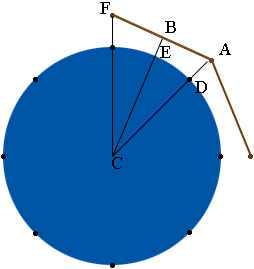SEARCH HOMEMath Central Quandaries & QueriesQuestion from Heath, a parent: I am building a fence around a water tank. the fence is to be in the shape of a normal octagon. The tank has a circumference of 57 ' 6''. I would like the fence to be 3 ft from the tank at the skinny point . How would I calculate(for the simple guy) where to set each of my 4x4 posts at the 8 corners. Any help would be greatly appreciated.Hi Heath,

I'm going to work in inches so the circumference of the tank is $57 \times 12 + 6 = 690$ inches. $690 / 8 = 86.25$ so I would start by marking 8 points on the circumference of the tank, 86.25 inches apart. The posts will be directly out from the tank at these positions.I have drawn the position of three of the posts and two of the eight fence sections. I want to look closely at the triangle $ABC$.

$CD$ and $CE$ are radii of the circular tank which has a circumference of 690 inches. The circumference of a circle is given by $2 \pi r$ where $r$ is the radius so

$2 \pi r = 690$

and hence

$r = \frac{690}{2 \pi} = 109.8 \mbox{ inches.}$

You told us that $|BE| = 36$ inches and hence $|BC| = 109.8 + 36 =145.8$ inches.

Angle $FCA$ measures $45$ degrees and hence angle $BCA$ measures $22.5$ degrees. Using some trigonometry $\cos(22.5) = \frac{|BC|}{|CA|}$ and hence

$|CA| = \frac{|BC|}{cos(22.5)} = \frac{145.85}{0.9239} = 157.8 \mbox{ inches.}$

$|CD| = 108.86$ inches so $|DA| = 157.8 - 108.86 = 49$ inches.

Go around the tank and measure out 49 inches from each of the 8 points you marked on the circumference of the tank. This should be the positions of the posts. There may be some error in the measurements so I would check the distance between the post positions before digging post holes. The distance between the posts, for example $|FA|$ should be consistent and you may need to do some fine tuning to guarantee this. My calculation give me that $|FA| = 120.8$ inches.

I hope this helps,
HarleyMath Central is supported by the University of Regina and The Pacific Institute for the Mathematical Sciences.